﻿ 基于Jointed Rock模型的不同裂隙形态页岩抗压强度研究 Study on the Compressive Strength of Shale with Different Fracture Morphology Based on Jointed Rock Model

Hans Journal of Civil Engineering
Vol. 08  No. 05 ( 2019 ), Article ID: 31362 , 12 pages
10.12677/HJCE.2019.85112

Study on the Compressive Strength of Shale with Different Fracture Morphology Based on Jointed Rock Model

Zhiqiang Huang*, Zhichao Fan#

College of Architecture and Environment, Sichuan University, Chengdu Sichuan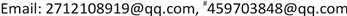Received: June 30th, 2019; accepted: July 15th, 2019; published: July 22nd, 2019ABSTRACT

As the main reservoir space and important migration channel of the shale gas, the fractures are frequently found in shale with a variety of distribution characteristics. The uniaxial and triaxial compression tests under different inclination angles of the non-fractured shale specimens were conducted, so as to obtain the shear strength parameters of foliation and matrix. Through the compression test data and previous literature data, it has shown that the Jointed Rock model is numerically suitable in calculating the rock which contains crack and foliation. The numerical simulation tests of different foliation dip angles and fracture inclination angle were conducted, it found that (1) for the different foliation angles, the influence of fracture angle on the compressive strength is similar, the influence of fracture inclination angle 0˚, 15˚, 90˚ on the compressive strength of shale can be neglected, the fracture inclination angles 30˚, 45˚, 60˚, 75˚ have great influence on the compressive strength and the degree of influence is 60˚ > 75˚ > 45˚ > 30˚. (2) The weaken degree of same fracture inclination angle on shale foliation dip angle 0˚, 90˚ compressive strength is approximately equal and influences slightly on 60˚. (3) The fracture weakening degree on the compressive strength of the shale is two-times-function relation with the fracture inclination angle, and the linear-function relation with the ratio of the compressive strength of the rock under the same confining pressure.

Keywords:Fractured Shale, Compression Test, Numerical Simulation, Compressive Strength1. 引言

2. 不同层理倾角下页岩压缩试验Table 1. Mechanical parameters of different angles of the shale foliation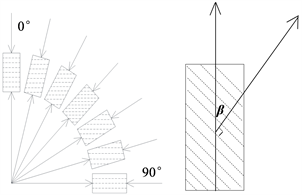Figure 1. The definition of angle of foliation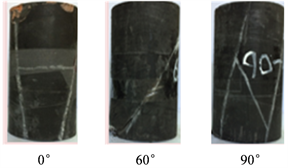Figure 2. Typical failure modes of different angles of shale foliation

Tien和Kuo等   借助层状岩石三轴试验数据给出了岩石弱面和基质的强度抗剪参数。同样，鉴于试件破坏形式，可以认为0˚、60˚试件的破坏分别受基质及层理的剪切强度控制。如图3，分别对0˚、60˚三轴压缩的抗压强度试验值进行线性拟合。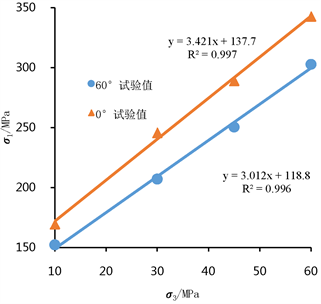Figure 3. Shale matrix and bedding strength parameters fitting

${\sigma }_{1,{0}^{\circ }}=b+m{\sigma }_{3}=137.72+3.421{\sigma }_{3}$ (1)

$\begin{array}{c}{\phi }_{b}=\mathrm{arcsin}\frac{m-1}{m+1}={33.20}^{\circ }\\ {c}_{b}=\frac{b\left(1-sin\phi \right)}{2cos\phi }=37.23\text{\hspace{0.17em}}\text{\hspace{0.17em}}\text{MPa}\end{array}\right\}$ (2)

${\sigma }_{1,{0}^{\circ }}=b+m{\sigma }_{3}=118.85+3.012{\sigma }_{3}$ (3)

$\begin{array}{c}{\phi }_{j}=\mathrm{arcsin}\frac{m-1}{m+1}={30.25}^{\circ }\\ {c}_{j}=\frac{b\left(1-sin\phi \right)}{2cos\phi }=34.14\text{\hspace{0.17em}}\text{MPa}\end{array}\right\}$ (4)

3. Jointed Rock模型应用验证

Jointed Rock模型考虑了含弱面岩体非均匀力学特性效应，该模型将含弱面岩体等价为各向同性岩石与各向异性面的组合，弱面的破坏特征由带拉伸判据的各向异性Mohr-Coulomb准则决定。在ANSYS17.0中，弱面位置是通过其法向向量w来定义的。假设最初弱面局部坐标系(u, v, w)与整体坐标系(x, y, z)吻合，首先将弱面沿z轴旋转角度α，然后将弱面以当前坐标系下的v轴旋转γ，弱面经过上述2次旋转可表示实际岩石中任意位置，而旋转之后弱面的法线方向为，

$w={\left[\begin{array}{ccc}\text{cos}\alpha \text{sin}\gamma & -\text{sin}\alpha \text{sin}\gamma & \text{cos}\gamma \end{array}\right]}^{\text{T}}$ (5)

ANSYS17.0中的Jointed Rock模型并不要求在试件的几何模型上对层理和基质进行区分，而是在定义材料非线性力学特征的过程中独立设置两者的强度参数等信息 。该模型将页岩视为一种均匀各向异性材料，不体现层理具体空间位置及厚度。层理及裂隙常常都被当作力学特性较弱的结构面统一对待，事实上两者有一定区别：层理与基质的区分主要表现在矿物的成分及排布方式上的差异，两者方向一致交互叠加；而天然裂隙与基质(层理)具有明显的分界线，裂隙宽度0.6~2 mm，方向随机 。因此有必要分别对Jointed Rock模型在层理及裂隙岩石上的适用性加以验证。

3.1. Jointed Rock模型在裂隙岩体中的应用验证

Ghazvinian等  人开展了预制裂隙面的物理模型材料试验，得到了多种工况下试件的抗压强度值。文献  中无裂隙试件的弹性模量及泊松比分别为1.43 GPa和0.31；无裂隙试件的粘结力及摩擦角分别为1.6 MPa和39.5˚。对4组不同形态的含裂隙试件进行了6种不同围压(0.5 MPa，1 MPa等)下的压缩试验，研究结果表明，当裂隙倾角θ为0˚时试件发生剪切及劈裂破坏，30˚时试件发生裂隙滑移破坏，15˚及45˚时发生劈裂及扭转破坏。Table 2. Compressive strength of different fracture angles under different confining pressures in Ref. 

${\sigma }_{1,{30}^{\circ }}=b+m{\sigma }_{3}=1.066+4.945{\sigma }_{3}$ (6)

$\begin{array}{c}{\phi }_{{30}^{\circ }}=\mathrm{arcsin}\frac{m-1}{m+1}={41.58}^{\circ }\\ {c}_{{30}^{\circ }}=\frac{b\left(1-\text{sin}\phi \right)}{2\text{cos}\phi }=0.24\text{\hspace{0.17em}}\text{MPa}\end{array}\right\}$ (7)

$\begin{array}{c}{\alpha }_{1}={\alpha }_{2}=0\\ {\gamma }_{1}=\theta \\ {\gamma }_{2}={90}^{\circ }+\theta \end{array}\right\}$ (8)Table 3. Numerical solution of different confining pressures (/MPa) in Ref. 

3.2. Jointed Rock模型在无裂隙页岩中的应用验证Table 4. Numerical solution (MPa) and deviation (%) of shale compressive strength

4. 不同裂隙倾角下页岩抗压强度的数值计算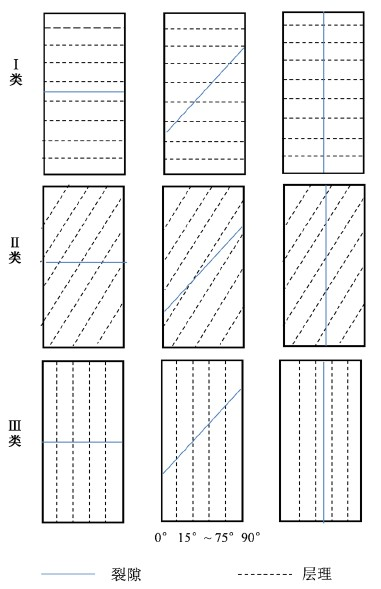Figure 4. Typical configurations of bedding and fractureTable 5. Numerical simulation of the strength parameters

${A}_{e}=\frac{{\sigma }_{1,\beta }-{\sigma }_{1,\beta \theta }}{{\sigma }_{1,\beta }}$ (9)Table 6. Ae (%) of Class I model under different calculation conditionsTable 7. Ae (%) of Class II model under different calculation conditionsTable 8. Ae (%) of Class III model under different calculation conditions

Ae随裂隙倾角θ的增大呈现先增后减的趋势，并在θ = 45˚~60˚内取得最大值，将表6中数据进行拟合得图6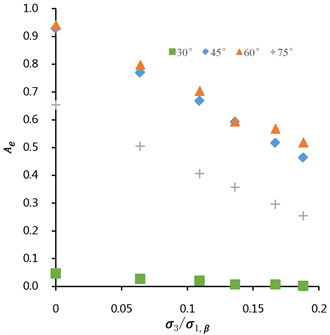Figure 5. The relationship between Ae and ${\sigma }_{3}/{\sigma }_{1,\beta }$ in model I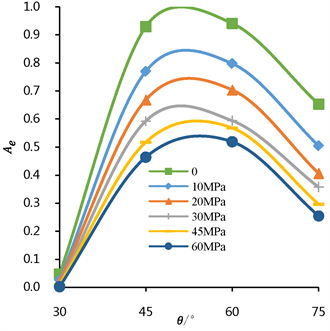Figure 6. The relationship between Ae and θ in model I

${\sigma }_{3}/{\sigma }_{1,\beta }$ 和θ为变量对Ae进行拟合，得到拟合关系式为式(10)，其调和确定系数 ${R}^{2}=0.9326$

${A}_{e}=-1.729\frac{{\sigma }_{3}}{{\sigma }_{1,\beta }}-3.331{\theta }^{2}+6.567\theta -2.299$ (10)

${A}_{e}=\left\{\begin{array}{l}-1.729\frac{{\sigma }_{3}}{{\sigma }_{1,\beta }}-3.331{\theta }^{2}+6.567\theta -2.2993\text{\hspace{0.17em}}\text{\hspace{0.17em}}\text{\hspace{0.17em}}\text{\hspace{0.17em}}\text{\hspace{0.17em}}{0}^{\circ }\le \theta \le {75}^{\circ }\\ 0\text{\hspace{0.17em}}\text{\hspace{0.17em}}\text{\hspace{0.17em}}\text{\hspace{0.17em}}\text{\hspace{0.17em}}\text{\hspace{0.17em}}\text{\hspace{0.17em}}\text{\hspace{0.17em}}\text{\hspace{0.17em}}\text{\hspace{0.17em}}\text{\hspace{0.17em}}\text{\hspace{0.17em}}\text{\hspace{0.17em}}\text{\hspace{0.17em}}\text{\hspace{0.17em}}\text{\hspace{0.17em}}\text{\hspace{0.17em}}\text{\hspace{0.17em}}\text{\hspace{0.17em}}\text{\hspace{0.17em}}\text{\hspace{0.17em}}\text{\hspace{0.17em}}\text{\hspace{0.17em}}\text{\hspace{0.17em}}\text{\hspace{0.17em}}\text{\hspace{0.17em}}\text{\hspace{0.17em}}\text{\hspace{0.17em}}\text{\hspace{0.17em}}\text{\hspace{0.17em}}\text{\hspace{0.17em}}\text{\hspace{0.17em}}\text{\hspace{0.17em}}\text{\hspace{0.17em}}\text{\hspace{0.17em}}\text{\hspace{0.17em}}\text{\hspace{0.17em}}\text{\hspace{0.17em}}\text{\hspace{0.17em}}\text{\hspace{0.17em}}\text{\hspace{0.17em}}\text{\hspace{0.17em}}\text{\hspace{0.17em}}\text{\hspace{0.17em}}\text{\hspace{0.17em}}\text{\hspace{0.17em}}\text{\hspace{0.17em}}\text{\hspace{0.17em}}\text{\hspace{0.17em}}\text{\hspace{0.17em}}\text{\hspace{0.17em}}\text{\hspace{0.17em}}\text{\hspace{0.17em}}\text{\hspace{0.17em}}\text{\hspace{0.17em}}\text{\hspace{0.17em}}\theta \le {30}^{\circ };{75}^{\circ }\le \theta \end{array}$ (11)

$\frac{\partial {A}_{e}}{\partial \theta }=-6.662\theta +6.567=0$ (12)

$\theta ={56.56}^{\circ }$ (13)

${A}_{e}=a\frac{{\sigma }_{3}}{{\sigma }_{1,\beta }}+b{\theta }^{2}+c\theta +d$ (14)Table 9. Summary of 3 kinds of model fitting parameter Ae

5. 结论

(1) 室内试验难以对裂隙页岩进行系统研究，借助ANSYS17.0 Jointed Rock模型可以对多种分布裂隙的页岩试件进行分析，具有明显的优势。

(2) 裂隙对不同层理倾角页岩抗压强度的影响趋势一致，57˚左右的裂隙倾角对页岩抗压强度的削弱程度最明显，之后向两边递减，在0˚，15˚，90˚时裂隙对页岩抗压强度基本不存在影响。

(3) 相同条件下，裂隙对60˚页岩的抗压强度影响较小，对0˚、90˚页岩的抗压强度影响较大，而这主要与裂隙页岩的破坏形式有关。

(4) 围压越高，围箍效应越明显，裂隙页岩抗压强度越大。另外，较高围压下试件沿裂隙方向的剪切滑移破坏难于发生，从而表现为裂隙对页岩抗压强度的影响作用(Ae)削弱。

Study on the Compressive Strength of Shale with Different Fracture Morphology Based on Jointed Rock Model[J]. 土木工程, 2019, 08(05): 966-977. https://doi.org/10.12677/HJCE.2019.85112

1. 1. 杨力行, 於文辉. “页岩气革命”为什么没有发生在中国[J]. 中国石油企业, 2013(3): 1-2.

2. 2. 王祥, 刘玉华, 张敏, 等. 页岩气形成条件及成藏影响因素研究[J]. 天然气地球科学, 2010, 21(2): 350-356.

3. 3. 聂海宽, 张金川. 页岩气储层类型和特征研究——以四川盆地及其周缘下古生界为例[J]. 石油实验地质, 2011, 33(3): 219-225+232.

4. 4. 周进举, 王德利. 含倾斜裂隙页岩介质地震波场传播特征研究[J]. 石油物探, 2014, 53(5): 501-508

5. 5. 魏元龙, 杨春和, 郭印同, 等. 单轴循环荷载下含天然裂隙脆性页岩变形及破裂特征试验研究[J]. 岩土力学, 2015, 36(6): 1649-1658.

6. 6. 王怡, 徐江, 梅春桂, 等. 含裂缝的硬脆性泥页岩理化及力学特性研究[J]. 石油天然气学报, 2011, 33(6): 104-108.

7. 7. 赵凯, 袁俊亮, 邓金根, 等. 层理产状对页岩气水平井井壁稳定性的影响[J]. 科学技术与工程, 2013, 13(3): 580-583.

8. 8. 康志勤, 赵阳升, 杨栋. 油页岩热破裂规律分形理论研究[J]. 岩石力学与工程学报, 2010, 29(1): 90-96.

9. 9. 梁榜, 梦吟, 蔡进. 泥页岩裂缝发育特征及其对含气性的影响[J]. 江汉石油科技, 2013, 23(4): 1-5.

10. 10. 林永学, 高书阳, 曾义金. 龙马溪组页岩强度评价与分析[J]. 石油钻探技术, 2015, 43(5): 20-25.

11. 11. Morgan, S.P. and Einstein, H.H. (2014) The Effect of Bedding Plane Orientation on Crack Propagation and Coalescence in Shale. In: 48th US Rock Mechanics/Geomechanics Symposium, Minneapoli, MN, 1-4 June 2014.

12. 12. Sun, K., Zhang, S. and Xin, L. (2016) Impacts of Bedding Directions of Shale Gas Reservoirs on Hydrau-lically Induced Crack Propagation. Natural Gas Industry B, 3, 139-145. https://doi.org/10.1016/j.ngib.2016.03.008

13. 13. Tien, Y.-M. and Kuo, M.-C. (2001) A Failure Criterion for Transversely Isotropic Rocks. International Journal of Rock Mechanics & Mining Sciences, 38, 399-412. https://doi.org/10.1016/S1365-1609(01)00007-7

14. 14. Kuo, M.-C., Tien, Y.-M. and Chu, C.A. (2004) Study of Failure Process and Failure Modes of Interstratified Rock Mass with an Emphasis on Specimen Preparation and Image Scanning. Gulf Rocks 2004, the 6th North America Rock Mechanics Symposium (NARMS): Rock Mechanics across Borders and Disciplines, Houston, TX, 5-9 June 2004, 1-12.

15. 15. ANSYS (2015) ANSYS Mechanical APDL Theory Reference Release 17. ANSYS, Inc., Canonsburg, PA.

16. 16. 张士诚, 郭天魁, 周彤,等. 天然页岩压裂裂缝扩展机理试验[J]. 石油学报, 2014, 35(3): 496-503.

17. 17. Ghazvinian, A. and Hadei, M.R. (2012) Effect of Discontinuity Ori-entation and Confinement on the Strength of Jointed Anisotropic Rocks. International Journal of Rock Mechanics & Mining Sciences, 55, 117-124. https://doi.org/10.1016/j.ijrmms.2012.06.008

18. 18. 杨志鹏, 何柏, 谢凌志, 等. 基于巴西劈裂试验的页岩强度与破坏模式研究[J]. 岩土力学, 2015, 36(12): 3447-3455.

19. 19. Yong, M.T., Ming, C.K. and Charng, H.J. (2006) An Experimental Investigation of the Failure Mechanism of Simulated Transversely Isotropic Rocks. International Journal of Rock Mechanics & Mining Sciences, 43, 1163-1181. https://doi.org/10.1016/j.ijrmms.2006.03.011

20. NOTES

*第一作者。

#通讯作者。